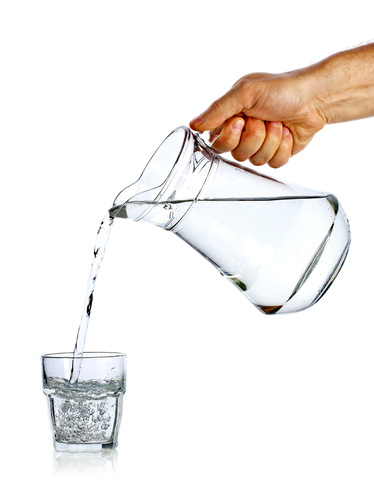# Convert Between Units of Capacity

In this worksheet, students will convert between units of capacity.Key stage:  KS 3

Curriculum topic:   Number

Curriculum subtopic:   Use Standard Units of Measure

Difficulty level:#### Worksheet Overview

What is capacity?Capacity is a measure of how much liquid something holds.

We often use capacities in everyday life. Some examples include quantities of ingredients in cooking, or amounts of fuel needed to fill our cars.

Can you think of any other examples where we would measure capacity?In the metric system, capacity is measured using litres or millilitres.

There are 1,000 ml in every litre.

Look at this diagram that you can use to help you convert between the two units:If we have a quantity measured in litres and we want to change it to ml, how do do we do this?

We want the arrow pointing from litres to ml, so we multiply by 1,000.

Example:

Change 4.3 l into ml:

4.3 x 1,000 = 4,300

So 4.3 l = 4,300 ml

And if we want to change a quantity from ml to litres? We're going the opposite way, so we divide by 1,000.

Example:

Change 620 ml into l:

620 ÷ 1,000 = 0.62

So 620 ml = 0.62 l